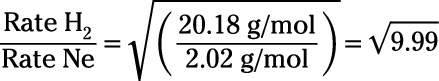##### Chemistry Workbook For Dummies with Online PracticeDifferent gases diffuse at different rates, depending on their molar masses. You can compare the rates at which two gases diffuse using Graham's law. Graham's law also applies to effusion, the process in which gas molecules flow through a small hole in a container.

Diffusion is the movement of a substance from an area of higher concentration to an area of lower concentration. Diffusion occurs spontaneously, on its own. It leads to mixing, eventually producing a homogenous mixture in which the concentration of any gaseous component is equal throughout an entire volume. Of course, that state of complete diffusion is an equilibrium state, and achieving equilibrium can take time.

Whether gases diffuse or effuse, they do so at a rate inversely proportional to the square root of their molar mass. In other words, more massive gas molecules diffuse and effuse more slowly than less massive gas molecules. So for Gases A and B, the following applies:Note: In the following problem, the molar masses are rounded to two decimal places before they are plugged into the formula.

By far, the most important part of solving effusion problems is identifying which gas you'll identify as Gas A and which gas you'll identify as Gas B when you plug your values into the equation. Don't switch them up!

## Solving an effusion problem

Now, on to the problem: How much faster does hydrogen gas effuse than neon gas?

Hydrogen gas refers to H2 because hydrogen is a diatomic element. Consult your periodic table (or your memory, if you're that good) to obtain the molar masses of hydrogen gas (2.02 g/mol) and neon gas (20.18 g/mol).

Finally, plug those values into the appropriate places within Graham's law, and you can see the ratio of effusion speed. In this example, let's assume that you chose hydrogen as Gas A and neon as Gas B.The answer you get to this problem is 3.16. Putting this number over 1 can help you understand your answer. The ratio 3.16/1 means is that for every 3.16 mol of hydrogen gas that effuses, 1.00 mol of neon gas will effuse. This ratio is designed to compare rates. So hydrogen gas effuses 3.16 times faster than neon.